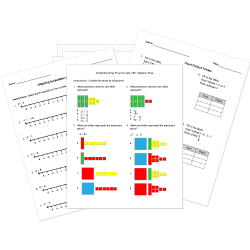Looking for Algebra worksheets?
Check out our pre-made Algebra worksheets!
 Tweet##### Browse Questions

You can create printable tests and worksheets from these Grade 6 Function and Algebra Concepts questions! Select one or more questions using the checkboxes above each question. Then click the add selected questions to a test button before moving to another page.

Previous Next
Evaluate.
$3^0 + 12$
1. 15
2. 13
3. 36
4. Impossible to complete
Grade 6 Linear Equations CCSS: 6.EE.B.7
What is x in this equation? 8x=48.
1. 8
2. 48
3. 6
4. none of the above
Grade 6 Algebraic Expressions CCSS: 6.EE.A.2, 6.EE.A.2a
3 times the quantity $m$ minus 7
1. $3(m-7)$
2. $3 xx m-7$
3. $3-m$
4. $3 xx m7$
Write 6 x 6 x 6 x 6 x 6 in exponential form.
1. $5^6$
2. $6^6$
3. $6^5$
4. $6^4$
Grade 6 Algebraic Expressions CCSS: 6.EE.A.2, 6.EE.A.2a
Write a verbal expression for the algebraic expression $4x+8$.
1. 4 more than 8 times a number x
2. 8 less than 4 times a number x
3. 8 more than 4 times a number x
4. 4 less than 8 times a number x
Grade 6 Algebraic Expressions CCSS: 6.EE.A.2, 6.EE.A.2a
two times a number plus 9
1. 2+5n
2. 2n+9
3. 29+n
4. 9n+2
Grade 6 Algebraic Expressions CCSS: 6.EE.A.2, 6.EE.A.2c
Grade 6 Linear Equations CCSS: 6.EE.B.7
1. $4^2$
2. $2^4$
3. $2^2$